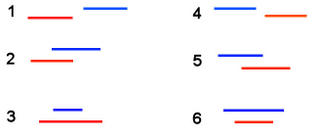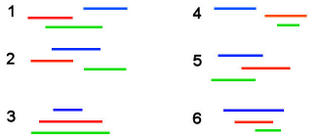## Sunday, September 18, 2005

### Combinatorial Diversion

I encountered a real-world problem recently, which turned out to be a fun little problem. In order to make the problem clearer, I've remapped the problem for everyone.

The image below shows two television programs, Red and Blue. We will assume that the starting/ending points of television programs cannot be shared. If that is the case, we have 6 ways to organize the programs relative to each other. We have the first case where Red begins and ends completely before Blue begins and ends. Another case is where Red begins, Blue begins during Red, Red ends during Blue, and Blue ends after Red is over. Yet another case is where Blue begins and ends completely within Red's scheduled block. We have another three relative configurations when we look at the other case where Red and Blue are reversed.So, the number of relative combinations for 1 television program and 2 television programs are f( 1 ) = 1 and f( 2 ) = 6. In general, what is f( N )?

Here are six different relative combinations for N = 3, just to help get the combinatorial juices flowing. Because I received some questions about the following combinations... let me state that the following is NOT complete for N = 3. There are way more than 6 relative combinations if you have 3 television programs.When I initially solved this problem, I did so in a rather inefficient manner. I ended up with a recurrence relation. However, the closed form answer is very clean and makes a lot of sense. Give it a try.

#### 1 comment:

Brute Force said...

JDot got it right. He gave me the cleaner solution...

Clean Solution:

F(N) = (2N)!/2^N

Recursive Solution:

F(N) = F(N-1) * N(2N-1)

Good work!